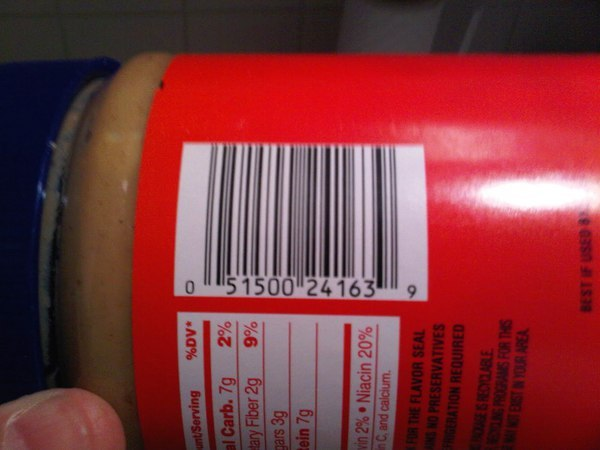``` # import the necessary packages
import numpy as np
import argparse
import cv2

# construct the argument parse and parse the arguments
ap = argparse.ArgumentParser()
ap.add_argument("-i", "--image", required = True, help = "path to the image file")
args = vars(ap.parse_args())
```

``` # load the image and convert it to grayscale
gray = cv2.cvtColor(image, cv2.COLOR_BGR2GRAY)

# compute the Scharr gradient magnitude representation of the images
# in both the x and y direction
gradX = cv2.Sobel(gray, ddepth = cv2.cv.CV_32F, dx = 1, dy = 0, ksize = -1)
gradY = cv2.Sobel(gray, ddepth = cv2.cv.CV_32F, dx = 0, dy = 1, ksize = -1)

```

2~3行：从磁盘载入图像并转换为灰度图。

7~8行：使用Scharr操作（指定使用ksize = -1）构造灰度图在水平和竖直方向上的梯度幅值表示。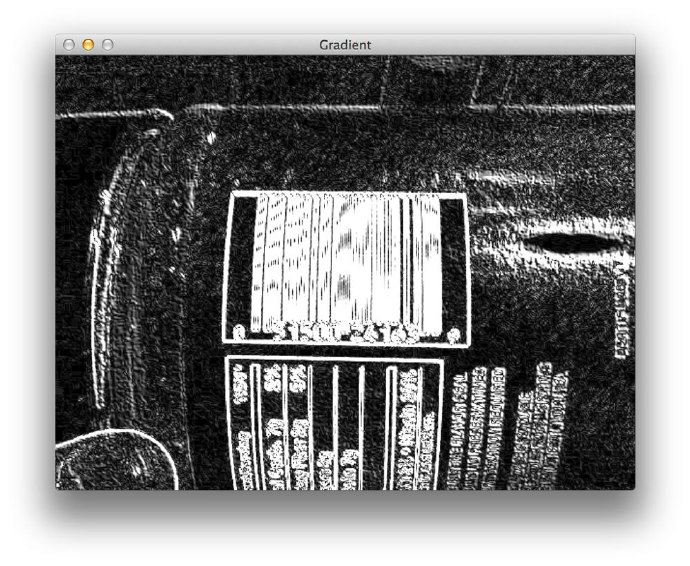``` # blur and threshold the image
(_, thresh) = cv2.threshold(blurred, 225, 255, cv2.THRESH_BINARY)
```

2行：我们要做的第一件事是使用9*9的内核对梯度图进行平均模糊，这将有助于平滑梯度表征的图形中的高频噪声。

3行：然后我们将模糊化后的图形进行二值化，梯度图中任何小于等于255的像素设为0（黑色），其余设为255（白色）。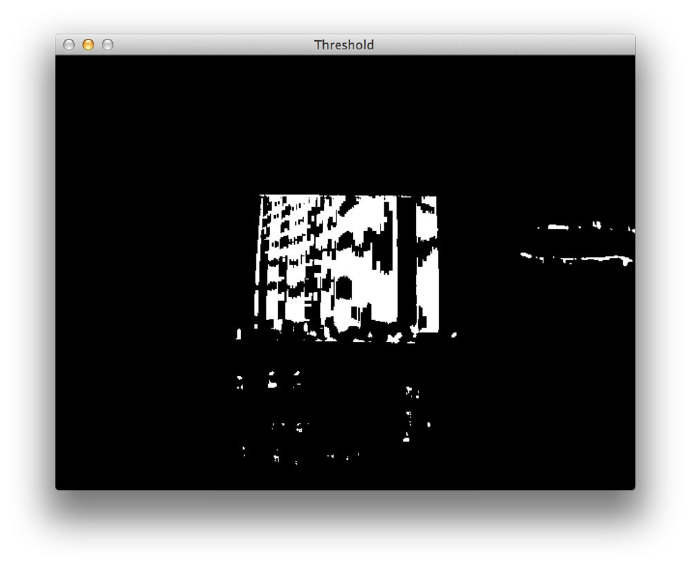``` # construct a closing kernel and apply it to the thresholded image
kernel = cv2.getStructuringElement(cv2.MORPH_RECT, (21, 7))
closed = cv2.morphologyEx(thresh, cv2.MORPH_CLOSE, kernel)
```

2行：我们首先使用cv2.getStructuringElement构造一个长方形内核。这个内核的宽度大于长度，因此我们可以消除条形码中垂直条之间的缝隙。

3行：这里进行形态学操作，将上一步得到的内核应用到我们的二值图中，以此来消除竖杠间的缝隙。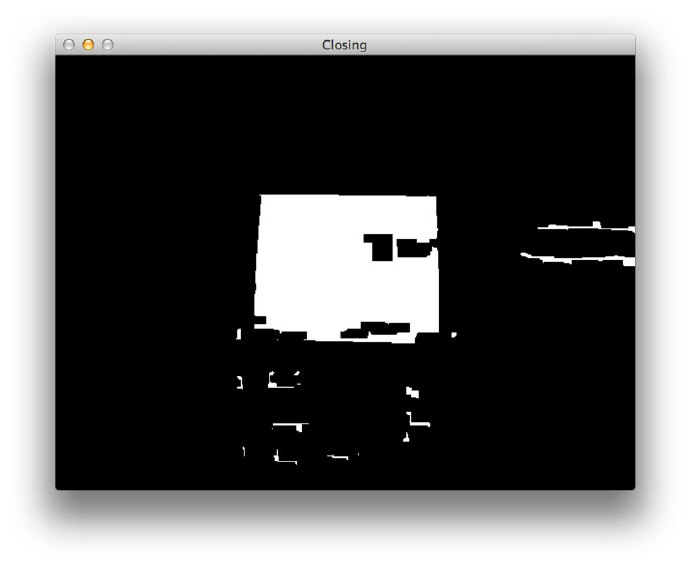``` # perform a series of erosions and dilations
closed = cv2.erode(closed, None, iterations = 4)
closed = cv2.dilate(closed, None, iterations = 4)
```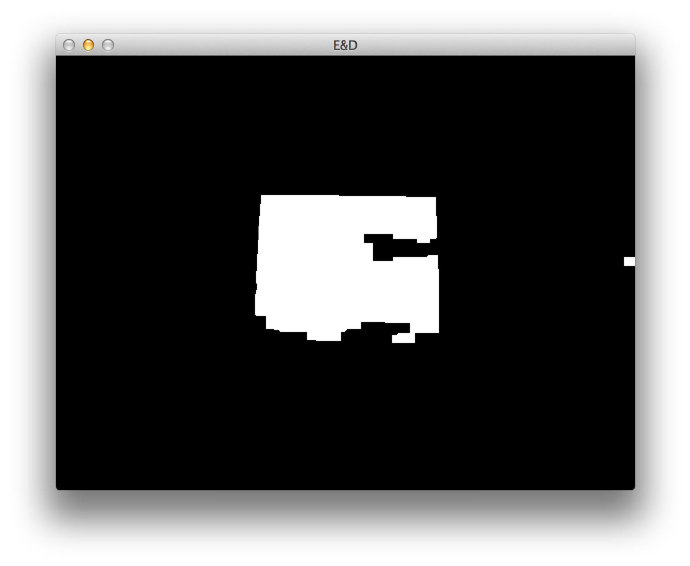``` # find the contours in the thresholded image, then sort the contours
# by their area, keeping only the largest one
(cnts, _) = cv2.findContours(closed.copy(), cv2.RETR_EXTERNAL,
cv2.CHAIN_APPROX_SIMPLE)
c = sorted(cnts, key = cv2.contourArea, reverse = True)

# compute the rotated bounding box of the largest contour
rect = cv2.minAreaRect(c)
box = np.int0(cv2.cv.BoxPoints(rect))

# draw a bounding box arounded the detected barcode and display the
# image
cv2.drawContours(image, [box], -1, (0, 255, 0), 3)
cv2.imshow("Image", image)
cv2.waitKey(0)
```

3~5行：幸运的是这一部分比较容易，我们简单地找到图像中的最大轮廓，如果我们正确完成了图像处理步骤，这里应该对应于条形码区域。

8~9行：然后我们为最大轮廓确定最小边框

13~15行：最后显示检测到的条形码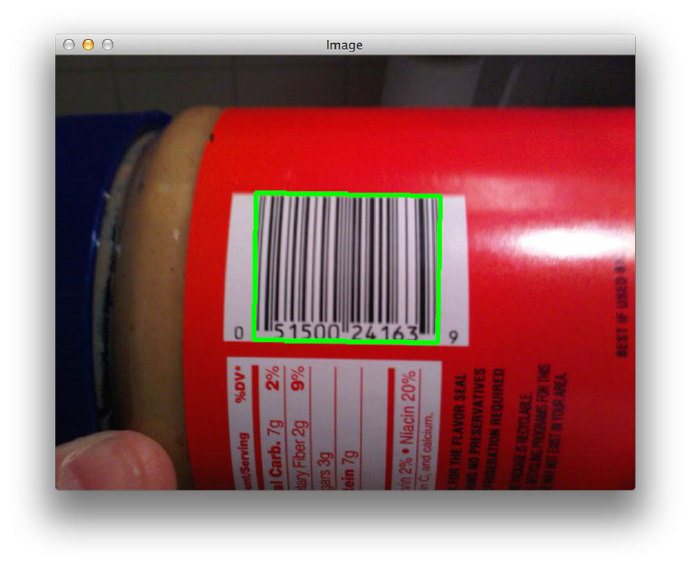```\$ python detect_barcode.py --image images/barcode_02.jpg
```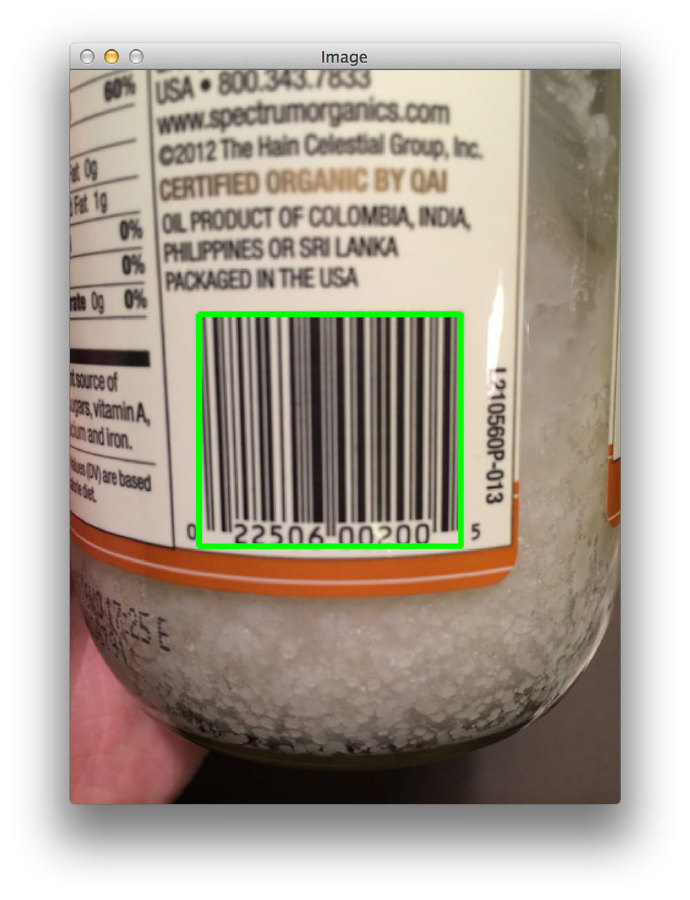```\$ python detect_barcode.py --image images/barcode_03.jpg
```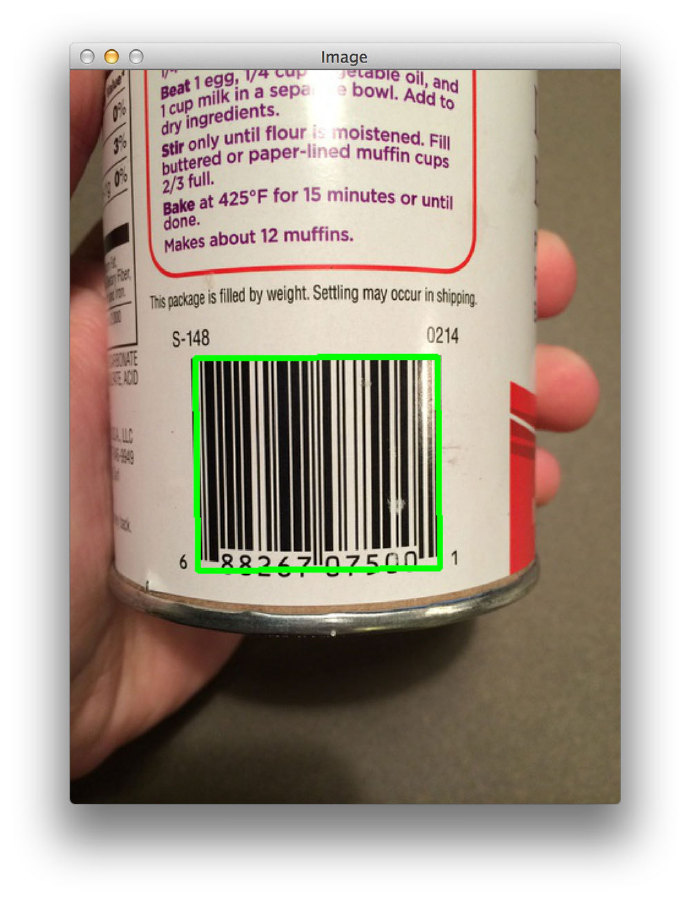```\$ python detect_barcode.py --image images/barcode_04.jpg
```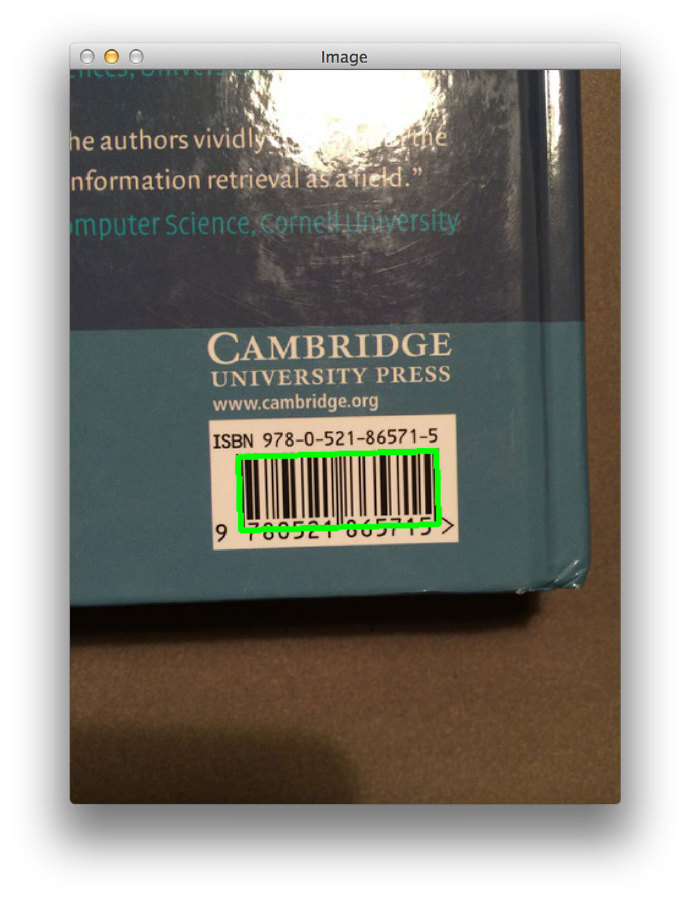```\$ python detect_barcode.py --image images/barcode_05.jpg
```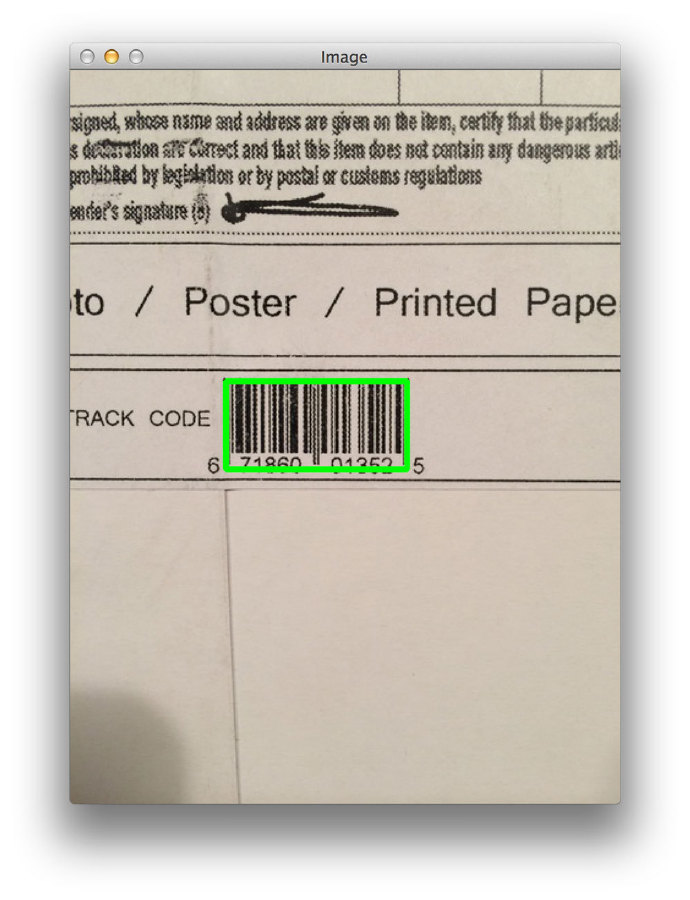```\$ python detect_barcode.py --image images/barcode_06.jpg
```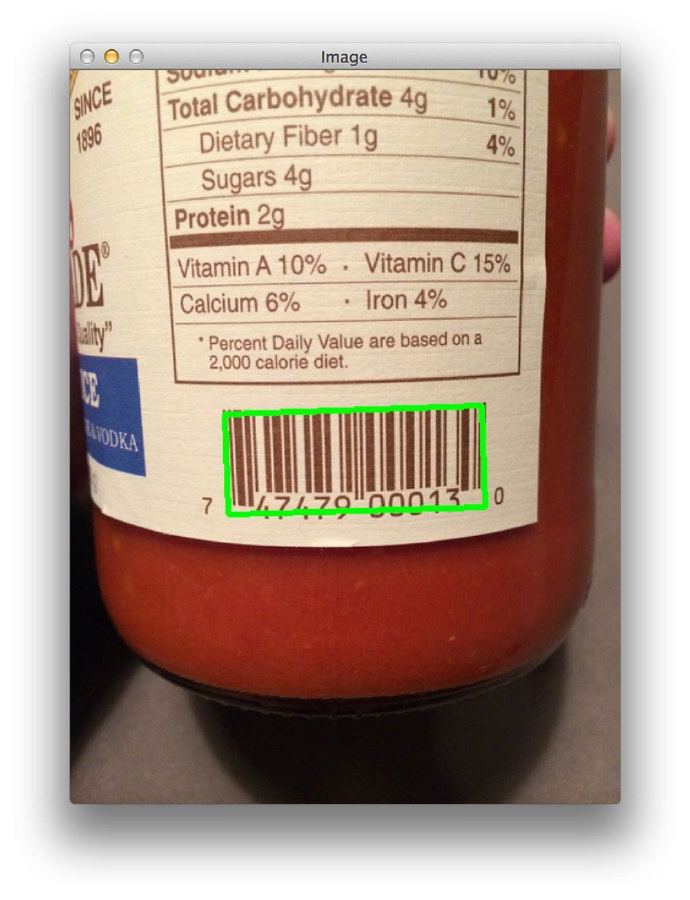1. 计算x方向和y方向上的Scharr梯度幅值表示